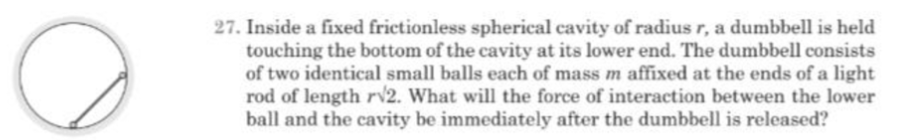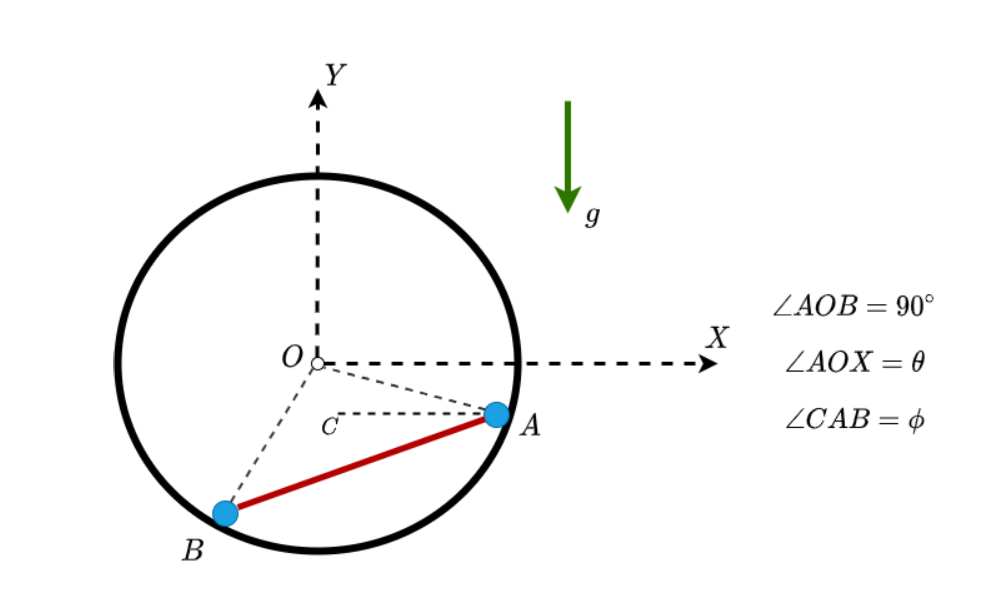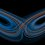# Dumbbell In a Cylindrical Cavity

Consider the following problem:At any general time $t$, the configuration of the system is as shown in the figure below:• Let the reaction force on the mass $A$ be $N_1$. It is directed along $AO$ and towards $O$.

• Let the reaction force on the mass $B$ be $N_2$. It is directed along $BO$ and towards $O$.

• Let the tension developed on the massless rod be $T$. The tension acts on mass $A$ along $AB$ directed towards $B$ and acts on mass $B$ along $BA$ directed towards $A$.

From the diagram, it is apparent that:

$\phi + \theta = \frac{\pi}{4}$

Let the coordinates of point mass $A$ be:

$x_A = r \cos{\theta}$ $y_A = -r\sin{\theta}$

Let the coordinates of point mass $B$ be:

$x_B = -r\sin{\theta}$ $y_B = -r\cos{\theta}$

The following equations can be obtained by drawing appropriate free body diagrams for each mass. I have left out the free body diagrams from this analysis.

$m\ddot{x}_A = -N_1 \cos{\theta} - T\cos{\phi}$ $m\ddot{y}_A = N_1 \sin{\theta}-mg - T\sin{\phi}$ $m\ddot{x}_B = N_2 \sin{\theta} + T\cos{\phi}$ $m\ddot{y}_B = N_2 \cos{\theta}-mg + T\sin{\phi}$

The acceleration expressions can be found by differentiating $x_A$, $y_A$, $x_B$ and $y_B$ twice with respect to time. Note that the system is released from rest, so:

$\theta(0) = 0$ $\dot{\theta}(0) = 0$

And let:

$\ddot{\theta}(0) = \alpha$

Also, when the rod is just released, one can compute the following results:

$\phi = \frac{\pi}{4}$ $\ddot{x}_A = 0$ $\ddot{y}_A = - r \alpha$ $\ddot{x}_B = -r\alpha$ $\ddot{y}_B = 0$

Plugging these all these expressions into the equations above, and simplifying gives:

$0 = -N_1 -\frac{T}{\sqrt{2}}$ $- r \alpha = -mg -\frac{T}{\sqrt{2}}$ $- r \alpha = \frac{T}{\sqrt{2}}$ $0 = \frac{T}{\sqrt{2} }+ N_2 - mg$

Solving for $N_2$ gives:

$\boxed{N_2 = \frac{3mg}{2}}$

The above is the reaction force computed when the system is just released from rest.Note by Karan Chatrath
8 months, 1 week ago

This discussion board is a place to discuss our Daily Challenges and the math and science related to those challenges. Explanations are more than just a solution — they should explain the steps and thinking strategies that you used to obtain the solution. Comments should further the discussion of math and science.

When posting on Brilliant:

• Use the emojis to react to an explanation, whether you're congratulating a job well done , or just really confused .
• Ask specific questions about the challenge or the steps in somebody's explanation. Well-posed questions can add a lot to the discussion, but posting "I don't understand!" doesn't help anyone.
• Try to contribute something new to the discussion, whether it is an extension, generalization or other idea related to the challenge.
• Stay on topic — we're all here to learn more about math and science, not to hear about your favorite get-rich-quick scheme or current world events.

MarkdownAppears as
*italics* or _italics_ italics
**bold** or __bold__ bold
- bulleted- list
• bulleted
• list
1. numbered2. list
1. numbered
2. list
Note: you must add a full line of space before and after lists for them to show up correctly
paragraph 1paragraph 2

paragraph 1

paragraph 2

[example link](https://brilliant.org)example link
> This is a quote
This is a quote
    # I indented these lines
# 4 spaces, and now they show
# up as a code block.

print "hello world"
# I indented these lines
# 4 spaces, and now they show
# up as a code block.

print "hello world"
MathAppears as
Remember to wrap math in $$ ... $$ or $ ... $ to ensure proper formatting.
2 \times 3 $2 \times 3$
2^{34} $2^{34}$
a_{i-1} $a_{i-1}$
\frac{2}{3} $\frac{2}{3}$
\sqrt{2} $\sqrt{2}$
\sum_{i=1}^3 $\sum_{i=1}^3$
\sin \theta $\sin \theta$
\boxed{123} $\boxed{123}$

## Comments

Sort by:

Top Newest

@Talulah Riley Here you go.

- 8 months, 1 week ago

Log in to reply

Nice solution. I've responded to your report btw; I don't know if you got a notification for the response @Karan Chatrath

- 8 months, 1 week ago

Log in to reply

@Karan Chatrath Thank you so much for the note.

- 8 months, 1 week ago

Log in to reply

@Karan Chatrath About the report, I solved the original Irodov problem (which doesn't have friction) correctly using energy conservation; but solving for tension numerically as per the equation above gives a tension of 800 Newtons at maximum.

- 8 months, 1 week ago

Log in to reply

@Karan Chatrath I've reposted the problem now.

- 8 months, 1 week ago

Log in to reply

×

Problem Loading...

Note Loading...

Set Loading...